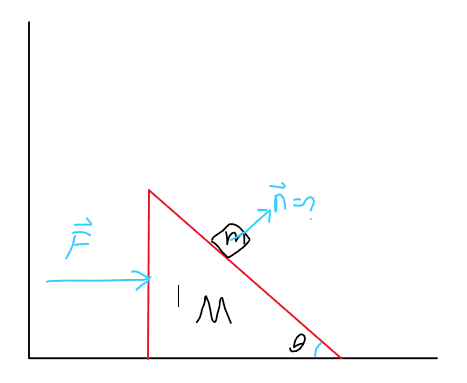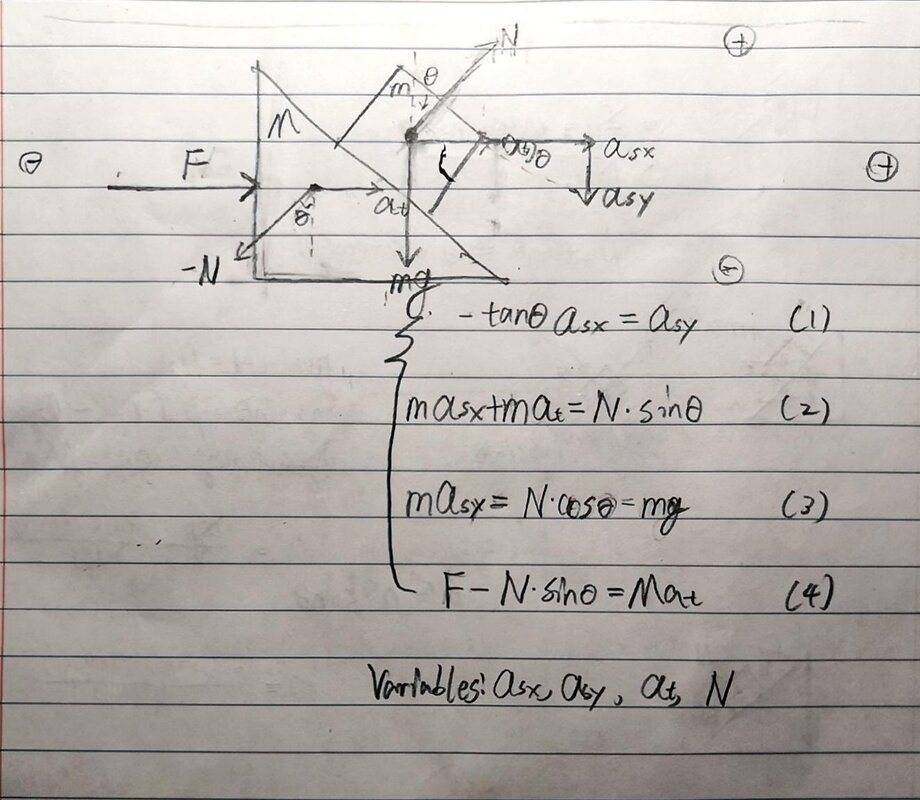# Normal force acting on a block on an accelerating wedge

Gold Member
Homework Statement:
I created this stupid question to help me better understand Newtonian mechanics.
Relevant Equations:
N/AWe have a wedge whose surface is ##\theta## from the horizontal surface. After a block is placed on its frictionless slant surface, the wedge starts to accelerate due to a force F. What is the normal force acting upon the block?

I have been trying to solve it but I got no clue. Could someone give me a hint?

## Answers and Replies

haruspex
Science Advisor
Homework Helper
Gold Member
2020 Award
First step: draw separate FBDs for the two components, showing forces. Next, create variables for the accelerations. Third, write the ΣF=ma equations and any appropriate kinematic equations that express relationships between the accelerations.
For that last, you need to represent the fact that the block stays on the wedge surface.

•Leo Liu
Gold Member
First step: draw separate FBDs for the two components, showing forces. Next, create variables for the accelerations. Third, write the ΣF=ma equations and any appropriate kinematic equations that express relationships between the accelerations.
For that last, you need to represent the fact that the block stays on the wedge surface.

Hi. The constraints in this problem gives these four distinct equations. Could you tell me if they are correct?Answer:
$$\begin{pmatrix} a_{sx}\\a_{sy}\\a_t\\N \end{pmatrix}=\begin{pmatrix} 0\\0\\-mg\\F \end{pmatrix}\begin{pmatrix} \tan \theta & 1 & 0 & 0\\ m & 0 & m & -sin \theta\\ 0 & m & 0 & -\cos \theta\\ 0 & 0 & M & \sin \theta \end{pmatrix}^{-1}$$

Last edited:
haruspex
Science Advisor
Homework Helper
Gold Member
2020 Award
Is asx the horizontal acceleration of the block in the lab frame or in the frame of the wedge?

If you define the height and leg of the wedge as ##h## and ##\ell##, then the net force on the block in the wedge's reference frame is in the direction of ##(\ell, -h)##. Since we know that the net force's norm is ##g\sin\theta##, we can describe it by ##\vec f_{b|w} = \frac{g\sin\theta}{\sqrt{\ell^2+h^2}}(\ell, -h)##. I think that this might work (you can find the block's absolute acceleration from this since you know the wedge's acceleration).$$\vec a_{b|w}=\vec a_b-\vec a_w$$The block starts falling from the top, so i think that it okay that we are considering the whole wedge to be only the topmost point (so that ##\vec x_b(0)-\vec x_w(0)=\vec y_b(0)-\vec y_w(0)=\vec 0##).

Last edited:
Gold Member
Is asx the horizontal acceleration of the block in the lab frame or in the frame of the wedge?
The frame of the wedge; since it is an non inertial frame, I added the acceleration of the frame to get the real acceleration.

haruspex
Science Advisor
Homework Helper
Gold Member
2020 Award
The frame of the wedge; since it is an non inertial frame, I added the acceleration of the frame to get the real acceleration.
Then it all looks fine.

•Leo Liu
Gold Member
the net force's norm is ##g\sin\theta##
Can you please tell me why this isn't ##\mu N##? I don't think you can use the component of the weight vector to calculate the sliding friction force.

Can you please tell me why this isn't ##\mu N##? I don't think you can use the component of the weight vector to calculate the sliding friction force.
Oh, that isn't the frictional force. You mentioned that your surface is frictionless, right? I meant by ##\vec f## the net force on the block.
Sorry, I shouldn't even have answered without reading your post well. I just assumed some things.The normal force would be in the direction of ##(\sin\theta,\cos\theta)## and with norm ##mg\cos\theta##, so:$$\vec N=mg\cos\theta(\sin\theta,\cos\theta)$$The force I have written in my other post has a missing ##m## factor. It should be:$$\vec f_{b|w}=mg\sin\theta(\cos\theta,-\sin\theta)$$

Gold Member
The normal force would be in the direction of ##(\sin\theta,\cos\theta)## and with norm ##mg\cos\theta##, so:$$\vec N=mg\cos\theta(\sin\theta,\cos\theta)$$The force I have written in my other post has a missing ##m## factor. It should be:$$\vec f_{b|w}=mg\sin\theta(\cos\theta,-\sin\theta)$$
I don't think this is the case since the wedge is accelerating.

I don't think this is the case since the wedge is accelerating.
Those are in the wedge's frame of reference. But yeah, I'm not sure if that relative acceleration formula in my first post is usable in this problem.

Please ignore my posts; the wedge is not an inertial frame of reference.